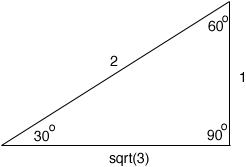Quandaries and Queries How do you find the length of the hypotenuse of a 30-60-90 triangle when the side opposite the 60 degree angle is 6 inches?   Amy Student Secondary Hi Amy, What I remember is that for a 30-60-90 triangle the sides are in the ratio of 1:2:sqrt(3) 2 is the largest of the three numbers so it is the length of the hypotenuse, and 1 is the smallest of the three numbers so it is the length of the side opposite the 30o angle.In your triangle the length of the side opposite the 60o angle is 6 inches. In my triangle the length of that side is sqrt(3) inches. Thus the sides in your triangle are 6/sqrt(3) times as large as the sides in my triangle. Hence your triangle has side lengths 16/sqrt(3) =  6/sqrt(3) 26/sqrt(3) = 12/sqrt(3) and sqrt(3)6/sqrt(3) = 6 Penny Go to Math Central